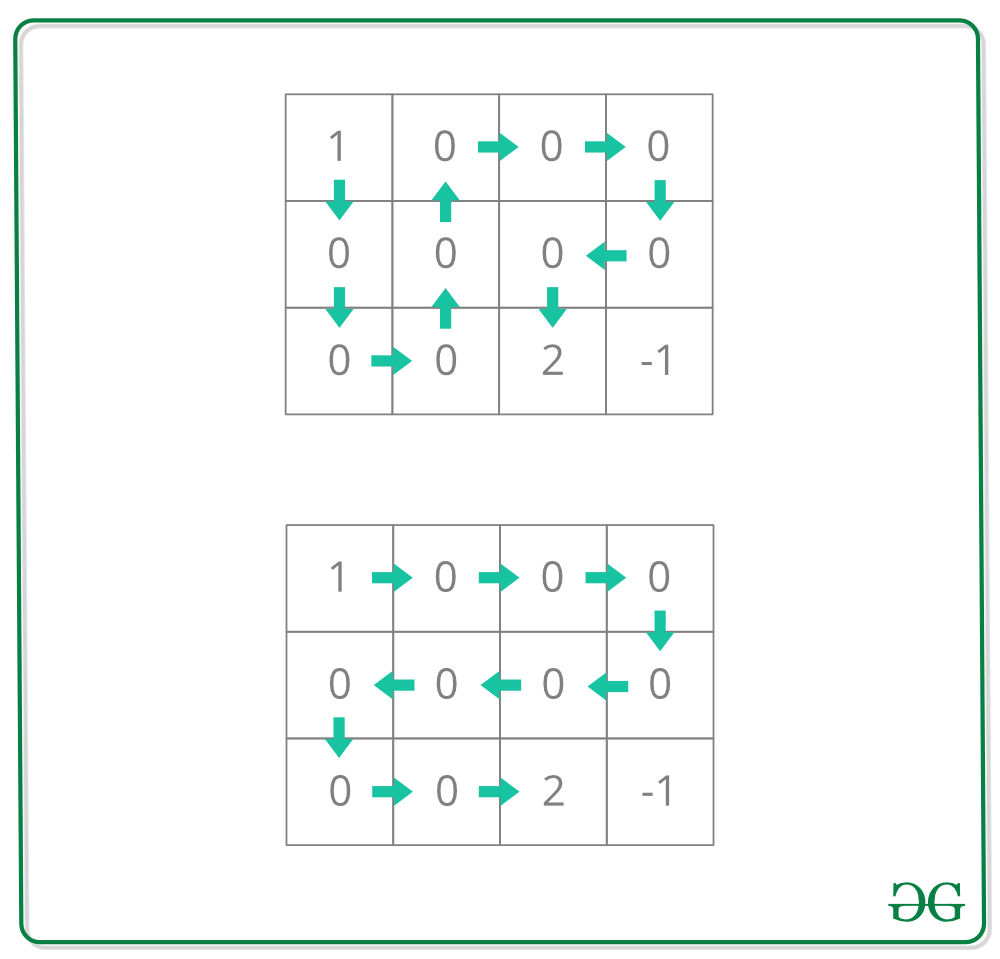# Unique paths covering every non-obstacle block exactly once in a grid

Given a grid grid[][] with 4 types of blocks:

• 1 represents the starting block. There is exactly one starting block.
• 2 represents the ending block. There is exactly one ending block.
• 0 represents empty block we can walk over.
• -1 represents obstacles that we cannot walk over.

The task is to count the number of paths from the starting block to the ending block such that every non-obstacle block is covered exactly once.

Examples:

Input: grid[][] = {
{1, 0, 0, 0},
{0, 0, 0, 0},
{0, 0, 2, -1} }
Output: 2
Following are the only paths covering all the non-obstacle blocks:Input: grid[][] = {
{1, 0, 0, 0},
{0, 0, 0, 0},
{0, 0, 0, 2} }
Output: 4

## Recommended: Please try your approach on {IDE} first, before moving on to the solution.

Approach: We can use simple DFS here with backtracking. We can check that a particular path has covered all the non-obstacle blocks by counting all the blocks encountered in the way and finally comparing it with the total number of blocks available and if they match, then we add it as a valid solution.

Below is the implementation of the above approach:

 `// C++ implementation of the approach ` `#include ` `using` `namespace` `std; ` ` `  `// Function for dfs. ` `// i, j ==> Current cell indexes ` `// vis ==> To mark visited cells ` `// ans ==> Result ` `// z ==> Current count 0s visited ` `// z_count ==> Total 0s present ` `void` `dfs(``int` `i, ``int` `j, vector >& grid, ` `         ``vector >& vis, ``int``& ans, ` `         ``int` `z, ``int` `z_count) ` `{ ` `    ``int` `n = grid.size(), m = grid.size(); ` ` `  `    ``// Mark the block as visited ` `    ``vis[i][j] = 1; ` `    ``if` `(grid[i][j] == 0) ` ` `  `        ``// update the count ` `        ``z++; ` ` `  `    ``// If end block reached ` `    ``if` `(grid[i][j] == 2) { ` ` `  `        ``// If path covered all the non- ` `        ``// obstacle blocks ` `        ``if` `(z == z_count) ` `            ``ans++; ` `        ``vis[i][j] = 0; ` `        ``return``; ` `    ``} ` ` `  `    ``// Up ` `    ``if` `(i >= 1 && !vis[i - 1][j] && grid[i - 1][j] != -1) ` `        ``dfs(i - 1, j, grid, vis, ans, z, z_count); ` ` `  `    ``// Down ` `    ``if` `(i < n - 1 && !vis[i + 1][j] && grid[i + 1][j] != -1) ` `        ``dfs(i + 1, j, grid, vis, ans, z, z_count); ` ` `  `    ``// Left ` `    ``if` `(j >= 1 && !vis[i][j - 1] && grid[i][j - 1] != -1) ` `        ``dfs(i, j - 1, grid, vis, ans, z, z_count); ` ` `  `    ``// Right ` `    ``if` `(j < m - 1 && !vis[i][j + 1] && grid[i][j + 1] != -1) ` `        ``dfs(i, j + 1, grid, vis, ans, z, z_count); ` ` `  `    ``// Unmark the block (unvisited) ` `    ``vis[i][j] = 0; ` `} ` ` `  `// Function to return the count of the unique paths ` `int` `uniquePaths(vector >& grid) ` `{ ` `    ``int` `z_count = 0; ``// Total 0s present ` `    ``int` `n = grid.size(), m = grid.size(); ` `    ``int` `ans = 0; ` `    ``vector > vis(n, vector<``bool``>(m, 0)); ` `    ``int` `x, y; ` `    ``for` `(``int` `i = 0; i < n; ++i) { ` `        ``for` `(``int` `j = 0; j < m; ++j) { ` ` `  `            ``// Count non-obstacle blocks ` `            ``if` `(grid[i][j] == 0) ` `                ``z_count++; ` `            ``else` `if` `(grid[i][j] == 1) { ` ` `  `                ``// Starting position ` `                ``x = i, y = j; ` `            ``} ` `        ``} ` `    ``} ` `    ``dfs(x, y, grid, vis, ans, 0, z_count); ` `    ``return` `ans; ` `} ` ` `  `// Driver code ` `int` `main() ` `{ ` `    ``vector > grid{ { 1, 0, 0, 0 }, ` `                               ``{ 0, 0, 0, 0 }, ` `                               ``{ 0, 0, 2, -1 } }; ` ` `  `    ``cout << uniquePaths(grid); ` `    ``return` `0; ` `} `

Output:

```2
```

Attention reader! Don’t stop learning now. Get hold of all the important DSA concepts with the DSA Self Paced Course at a student-friendly price and become industry ready.

My Personal Notes arrow_drop_upCheck out this Author's contributed articles.

If you like GeeksforGeeks and would like to contribute, you can also write an article using contribute.geeksforgeeks.org or mail your article to contribute@geeksforgeeks.org. See your article appearing on the GeeksforGeeks main page and help other Geeks.

Please Improve this article if you find anything incorrect by clicking on the "Improve Article" button below.